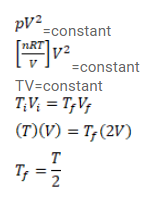# Use R=8.3 J/mol-K wherever required. During an experiment, an ideal gas is foundQuestion:

Use $R=8.3 \mathrm{~J} / \mathrm{mol}-\mathrm{K}$ wherever required.

During an experiment, an ideal gas is found to obey an additional law $p V^{2}=$ constant. The gas is initially at a temperature T and a volume V. Find the temperature when it expands to a volume $2 \mathrm{~V}$.

Solution: